Hits: 8

# B-tree

#### In this tutorial, you will learn what a B-tree is. Also, you will find working examples of search operation on a B-tree in Python.

B-tree is a special type of self-balancing search tree in which each node can contain more than one key and can have more than two children. It is a generalized form of the binary search tree.

It is also known as a height-balanced m-way tree.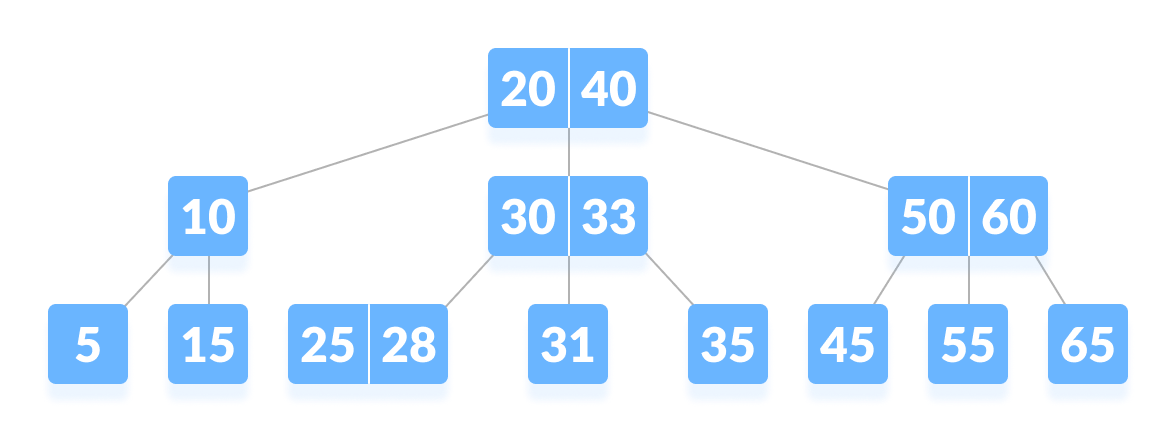B-tree

## Why B-tree?

The need for B-tree arose with the rise in the need for lesser time in accessing the physical storage media like a hard disk. The secondary storage devices are slower with a larger capacity. There was a need for such types of data structures that minimize the disk accesses.

Other data structures such as a binary search tree, avl tree, red-black tree, etc can store only one key in one node. If you have to store a large number of keys, then the height of such trees becomes very large and the access time increases.

However, B-tree can store many keys in a single node and can have multiple child nodes. This decreases the height significantly allowing faster disk accesses.

## B-tree Properties

1. For each node x, the keys are stored in increasing order.
2. In each node, there is a boolean value x.leaf which is true if x is a leaf.
3. If n is the order of the tree, each internal node can contain at most n – 1 keys along with a pointer to each child.
4. Each node except root can have at most n children and at least n/2 children.
5. All leaves have the same depth (i.e. height-h of the tree).
6. The root has at least 2 children and contains a minimum of 1 key.
7. If n ≥ 1, then for any n-key B-tree of height h and minimum degree `t ≥ 2``h ≥ logt (n+1)/2`.

## Operations

### Searching

Searching for an element in a B-tree is the generalized form of searching an element in a Binary Search Tree. The following steps are followed.

1. Starting from the root node, compare k with the first key of the node.
If `k = the first key of the node`, return the node and the index.
2. If `k.leaf = true`, return NULL (i.e. not found).
3. If `k < the first key of the root node`, search the left child of this key recursively.
4. If there is more than one key in the current node and `k > the first key`, compare k with the next key in the node.
If `k < next key`, search the left child of this key (ie. k lies in between the first and the second keys).
Else, search the right child of the key.
5. Repeat steps 1 to 4 until the leaf is reached.

### Searching Example

1. Let us search key `k = 17` in the tree below of degree 3.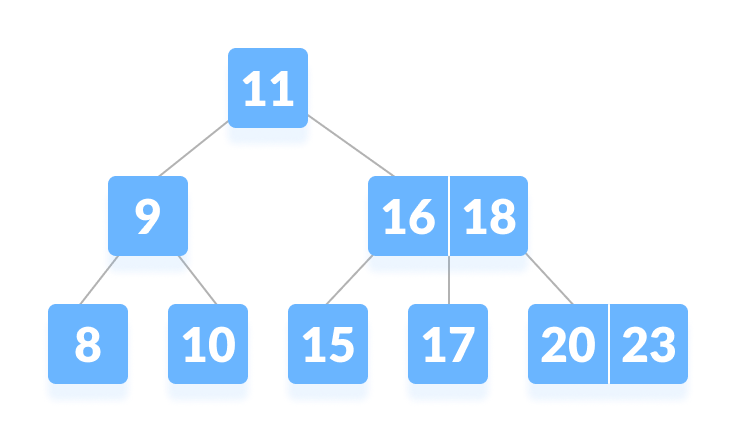B-tree
2. k is not found in the root so, compare it with the root key.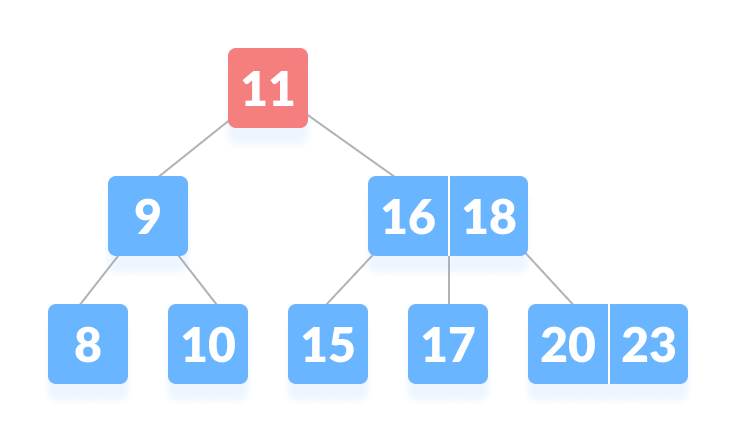k is not found on the root node
3. Since `k > 11`, go to the right child of the root node.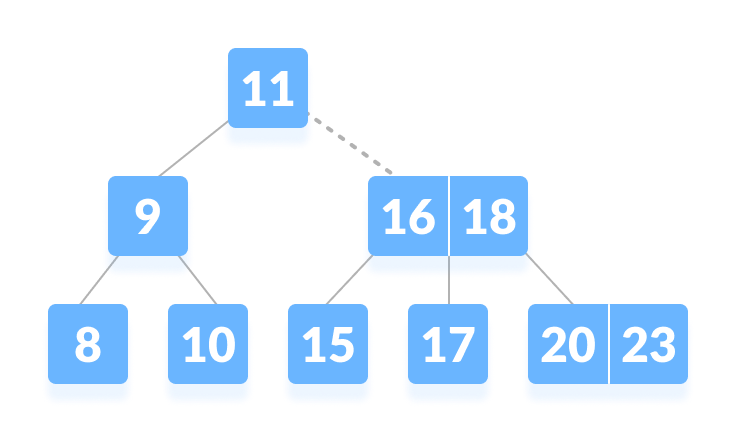Go to the right subtree
4. Compare k with 16. Since `k > 16`, compare k with the next key 18.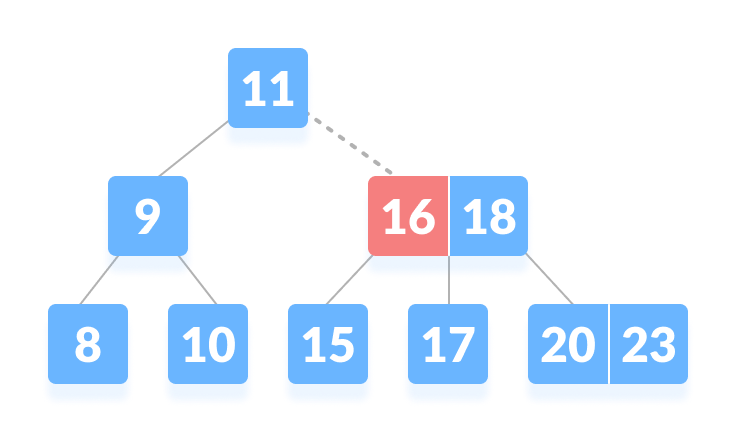Compare with the keys from left to right
5. Since `k < 18`, k lies between 16 and 18. Search in the right child of 16 or the left child of 18.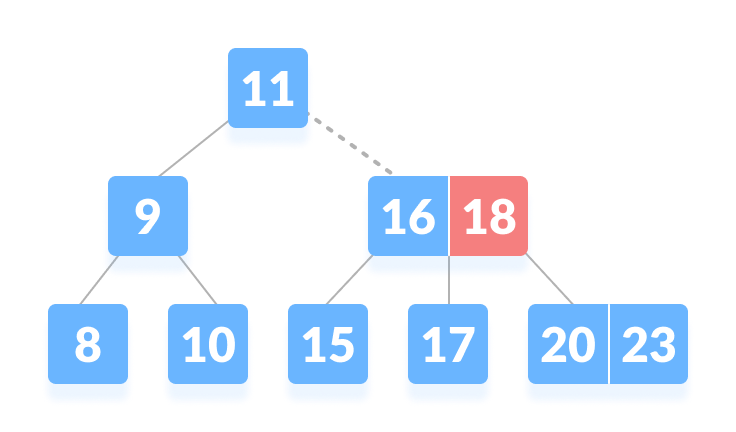k lies in between 16 and 18
6. k is found.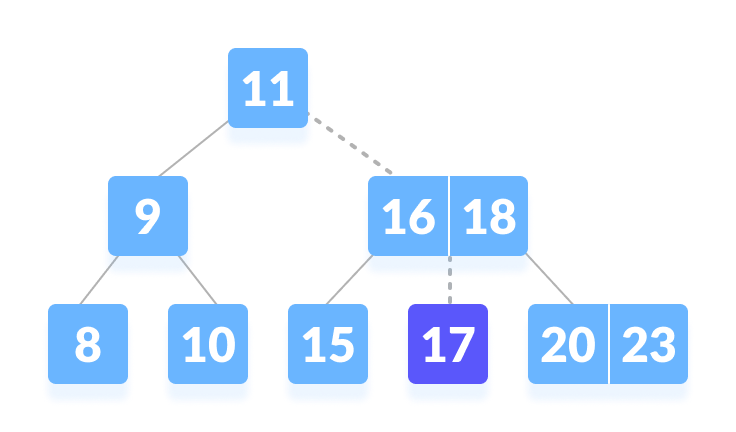k is found

## Algorithm for Searching an Element

``````BtreeSearch(x, k)
i = 1
while i ≤ n[x] and k ≥ keyi[x]        // n[x] means number of keys in x node
do i = i + 1
if i  n[x] and k = keyi[x]
then return (x, i)
if leaf [x]
then return NIL
else
return BtreeSearch(ci[x], k)
``````

To learn more about different B-tree operations, please visit

• Insertion on B-tree
• Deletion on B-tree

## Python Examples

``````/* Searching a key on a B-tree in Python */

/* Create node */
class BTreeNode:
def __init__(self, leaf=False):
self.leaf = leaf
self.keys = []
self.child = []

class BTree:
def __init__(self, t):
self.root = BTreeNode(True)
self.t = t

/* Print the tree */
def print_tree(self, x, l=0):
print("Level ", l, " ", len(x.keys), end=":")
for i in x.keys:
print(i, end=" ")
print()
l += 1
if len(x.child) > 0:
for i in x.child:
self.print_tree(i, l)

/* Search key */
def search_key(self, k, x=None):
if x is not None:
i = 0
while i < len(x.keys) and k > x.keys[i]:
i += 1
if i < len(x.keys) and k == x.keys[i]:
return (x, i)
elif x.leaf:
return None
else:
return self.search_key(k, x.child[i])
else:
return self.search_key(k, self.root)

/* Insert the key */
def insert_key(self, k):
root = self.root
if len(root.keys) == (2 * self.t) - 1:
temp = BTreeNode()
self.root = temp
temp.child.insert_key(0, root)
self.split(temp, 0)
self.insert_non_full(temp, k)
else:
self.insert_non_full(root, k)

/* Insert non full condition */
def insert_non_full(self, x, k):
i = len(x.keys) - 1
if x.leaf:
x.keys.append((None, None))
while i >= 0 and k < x.keys[i]:
x.keys[i + 1] = x.keys[i]
i -= 1
x.keys[i + 1] = k
else:
while i >= 0 and k < x.keys[i]:
i -= 1
i += 1
if len(x.child[i].keys) == (2 * self.t) - 1:
self.split(x, i)
if k > x.keys[i]:
i += 1
self.insert_non_full(x.child[i], k)

/* Split */
def split(self, x, i):
t = self.t
y = x.child[i]
z = BTreeNode(y.leaf)
x.child.insert_key(i + 1, z)
x.keys.insert_key(i, y.keys[t - 1])
z.keys = y.keys[t: (2 * t) - 1]
y.keys = y.keys[0: t - 1]
if not y.leaf:
z.child = y.child[t: 2 * t]
y.child = y.child[0: t - 1]

def main():
B = BTree(3)

for i in range(10):
B.insert_key((i, 2 * i))

B.print_tree(B.root)

if B.search_key(8) is not None:
print("nFound")
else:
print("nNot found")

if __name__ == '__main__':
main()``````

## Searching Complexity on B Tree

Worst case Time complexity: `Θ(log n)`

Average case Time complexity: `Θ(log n)`

Best case Time complexity: `Θ(log n)`

Average case Space complexity: `Θ(n)`

Worst case Space complexity: `Θ(n)`

## B Tree Applications

• databases and file systems
• to store blocks of data (secondary storage media)
• multilevel indexing

# Special 95% discount

## 2000+ Applied Machine Learning & Data Science Recipes

### Portfolio Projects for Aspiring Data Scientists: Tabular Text & Image Data Analytics as well as Time Series Forecasting in Python & R## Two Machine Learning Fields

There are two sides to machine learning:

• Practical Machine Learning:This is about querying databases, cleaning data, writing scripts to transform data and gluing algorithm and libraries together and writing custom code to squeeze reliable answers from data to satisfy difficult and ill defined questions. It’s the mess of reality.
• Theoretical Machine Learning: This is about math and abstraction and idealized scenarios and limits and beauty and informing what is possible. It is a whole lot neater and cleaner and removed from the mess of reality.

Data Science Resources: Data Science Recipes and Applied Machine Learning Recipes

Introduction to Applied Machine Learning & Data Science for Beginners, Business Analysts, Students, Researchers and Freelancers with Python & R Codes @ Western Australian Center for Applied Machine Learning & Data Science (WACAMLDS) !!!

Latest end-to-end Learn by Coding Recipes in Project-Based Learning:

Applied Statistics with R for Beginners and Business Professionals

Data Science and Machine Learning Projects in Python: Tabular Data Analytics

Data Science and Machine Learning Projects in R: Tabular Data Analytics

Python Machine Learning & Data Science Recipes: Learn by Coding

R Machine Learning & Data Science Recipes: Learn by Coding

Comparing Different Machine Learning Algorithms in Python for Classification (FREE)

`Disclaimer: The information and code presented within this recipe/tutorial is only for educational and coaching purposes for beginners and developers. Anyone can practice and apply the recipe/tutorial presented here, but the reader is taking full responsibility for his/her actions. The author (content curator) of this recipe (code / program) has made every effort to ensure the accuracy of the information was correct at time of publication. The author (content curator) does not assume and hereby disclaims any liability to any party for any loss, damage, or disruption caused by errors or omissions, whether such errors or omissions result from accident, negligence, or any other cause. The information presented here could also be found in public knowledge domains.  `